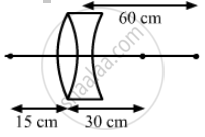Department of Pre-University Education, KarnatakaPUC Karnataka Science Class 12
Advertisement Remove all ads

# A Point Object is Placed at a Distance of 15 Cm from a Convex Lens. the Image is Formed on the Other Side at a Distance of 30 Cm from the Lens. - Physics

Sum

A point object is placed at a distance of 15 cm from a convex lens. The image is formed on the other side at a distance of 30 cm from the lens. When a concave lens is placed in contact with the convex lens, the image shifts away further by 30 cm. Calculate the focal lengths of the two lenses.

Advertisement Remove all ads

#### Solution

Given,
Distance between point object and convex lens, u = 15 cm
Distance between the image of the point object and convex lens, v = 30 cm
Let fc be the focal length of the convex lens.Then, using lens formula, we have:
$\frac{1}{v} - \frac{1}{u} = \frac{1}{f_c}$
$\Rightarrow \frac{1}{f_c} = \frac{1}{30} - \frac{1}{( - 15)}$
$\Rightarrow \frac{1}{f_c} = \frac{1}{30} + \frac{1}{15} = \frac{3}{30}$
$\Rightarrow f_c = 10 \text{ cm }$
Now, as per the question, the concave lens is placed in contact with the convex lens. So the image is shifted by a distance of 30 cm.
Again, let vf be the final image distance from concave lens, then:
$v_f$ = + (30 + 30) = + 60 cm
Object distance from the concave lens, = 30 cm
If fd is the focal length of concave lens then
Using lens formula, we have:
$\frac{1}{v_f} - \frac{1}{v} = \frac{1}{f_d}$
$\Rightarrow \frac{1}{f_d} = \frac{1}{60} - \frac{1}{30}$
$\Rightarrow \frac{1}{f_d} = \frac{30 - 60}{60 \times 30} = \frac{- 30}{60 \times 30}$
$\Rightarrow f_d = - 60 \text{ cm }$
Hence, the focal length (fc ) of convex lens is 10 cm and that of the concave lens (fd ) is 60 cm.

Concept: Refraction at Spherical Surfaces and by Lenses - Refraction at Spherical Surfaces
Is there an error in this question or solution?
Advertisement Remove all ads

#### APPEARS IN

HC Verma Class 11, 12 Concepts of Physics 1
Chapter 18 Geometrical Optics
Q 71 | Page 416
Advertisement Remove all ads

#### Video TutorialsVIEW ALL 

Advertisement Remove all ads
Share
Notifications

View all notifications

Forgot password?